Performs hierarchical/agglomerative clustering on the condensed distance matrix y.

y must be a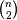sized vector where n is the number of original observations paired in the distance matrix. The behavior of this function is very similar to the MATLAB linkage function.

A 4 by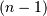matrix Z is returned. At the-th iteration, clusters with indices Z[i, 0] and Z[i, 1] are combined to form cluster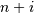. A cluster with an index less thancorresponds to one of theoriginal observations. The distance between clusters Z[i, 0] and Z[i, 1] is given by Z[i, 2]. The fourth value Z[i, 3] represents the number of original observations in the newly formed cluster.

The following linkage methods are used to compute the distance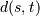between two clustersand. The algorithm begins with a forest of clusters that have yet to be used in the hierarchy being formed. When two clustersandfrom this forest are combined into a single cluster,andare removed from the forest, andis added to the forest. When only one cluster remains in the forest, the algorithm stops, and this cluster becomes the root.

A distance matrix is maintained at each iteration. The d[i,j] entry corresponds to the distance between clusterand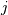in the original forest.

At each iteration, the algorithm must update the distance matrix to reflect the distance of the newly formed cluster u with the remaining clusters in the forest.

Suppose there are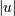original observations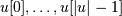in clusterand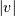original objects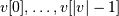in cluster. Recallandare combined to form cluster. Letbe any remaining cluster in the forest that is not.

The following are methods for calculating the distance between the newly formed clusterand each.

• method=’single’ assigns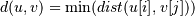for all pointsin clusterandin cluster. This is also known as the Nearest Point Algorithm.

• method=’complete’ assigns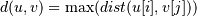for all pointsin cluster u andin cluster. This is also known by the Farthest Point Algorithm or Voor Hees Algorithm.

• method=’average’ assigns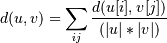for all pointsandwhereandare the cardinalities of clustersand, respectively. This is also called the UPGMA algorithm. This is called UPGMA.

• method=’weighted’ assigns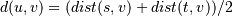where cluster u was formed with cluster s and t and v is a remaining cluster in the forest. (also called WPGMA)

• method=’centroid’ assigns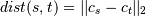where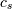and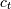are the centroids of clustersand, respectively. When two clustersandare combined into a new cluster, the new centroid is computed over all the original objects in clustersand. The distance then becomes the Euclidean distance between the centroid ofand the centroid of a remaining clusterin the forest. This is also known as the UPGMC algorithm.

• method=’median’ assigns math:d(s,t) like the centroid method. When two clustersandare combined into a new cluster, the average of centroids s and t give the new centroid. This is also known as the WPGMC algorithm.

• method=’ward’ uses the Ward variance minimization algorithm. The new entry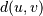is computed as follows,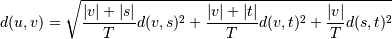whereis the newly joined cluster consisting of clustersand,is an unused cluster in the forest,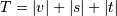, and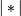is the cardinality of its argument. This is also known as the incremental algorithm.

Warning: When the minimum distance pair in the forest is chosen, there may be two or more pairs with the same minimum distance. This implementation may chose a different minimum than the MATLAB version.

Parameters: y : ndarray A condensed or redundant distance matrix. A condensed distance matrix is a flat array containing the upper triangular of the distance matrix. This is the form that pdist returns. Alternatively, a collection ofobservation vectors in n dimensions may be passed as anbyarray. method : str, optional The linkage algorithm to use. See the Linkage Methods section below for full descriptions. metric : str, optional The distance metric to use. See the distance.pdist function for a list of valid distance metrics. Z : ndarray The hierarchical clustering encoded as a linkage matrix.